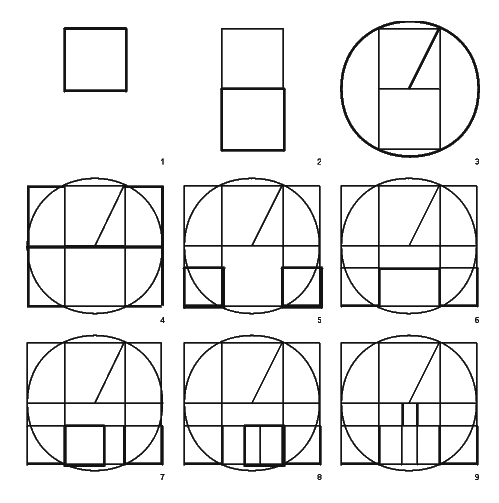Figures for Lionel March's
Palladio's Villa Emo: The Golden Proportion Hypothesis Rebutted

| Fig. 1 | Fig. 2 | Fig. 3 | Fig. 4 | Fig. 5 | Fig. 6 | Fig. 7 | Fig. 8 | Fig. 9 | Fig. 10 |
| Fig. 11 | Fig. 12 | Fig. 13 | Fig. 14 | Fig. 15 | Fig. 16 | Fig. 17 | Fig. 18 | Fig. 19 | Fig. 20 | Fig. 21 |

 Fig. 11a. The generation of the extreme and mean ration (EMR) scheme for Villa Emo:1) a square; 2) add a square to make a double square; 3) strike a circle to circumscribe the double square; 4) draw the diameter and extend the double square into a rectangle touching the circle; 5) draw two squares to produce the smaller EMR rectangles; 6) complete the EMR rectangle between the two squares; 6) complete the EMR rectangle between the two squares; 7) subtract a square from the left side of this rectangle; 8) subtract another square from the right side; 9) complete the small EMR rectangle in the center of the scheme. | go to Fig. 11b | back to text |

| Top of Page |